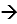# Problem: The Ksp of calcium carbonate, CaCO3, is 3.36 x 10-9. Calculate the solubility of this compound in g/L.

###### FREE Expert Solution

For this problem, we´re asked to calculate the solubility of this compound in g/L based on its Ksp of 3.36x10-9.

The compound is ionic and is a little soluble in water, with a dissociation as follows:

The Calcium ion Ca2+ is in group 2A and has a charge of +2. Carbonate then has a charge of -2:

CaCO3(s)Ca2+(aq) + CO32-(aq)

79% (441 ratings)###### Problem Details

The Ksp of calcium carbonate, CaCO3, is 3.36 x 10-9. Calculate the solubility of this compound in g/L.